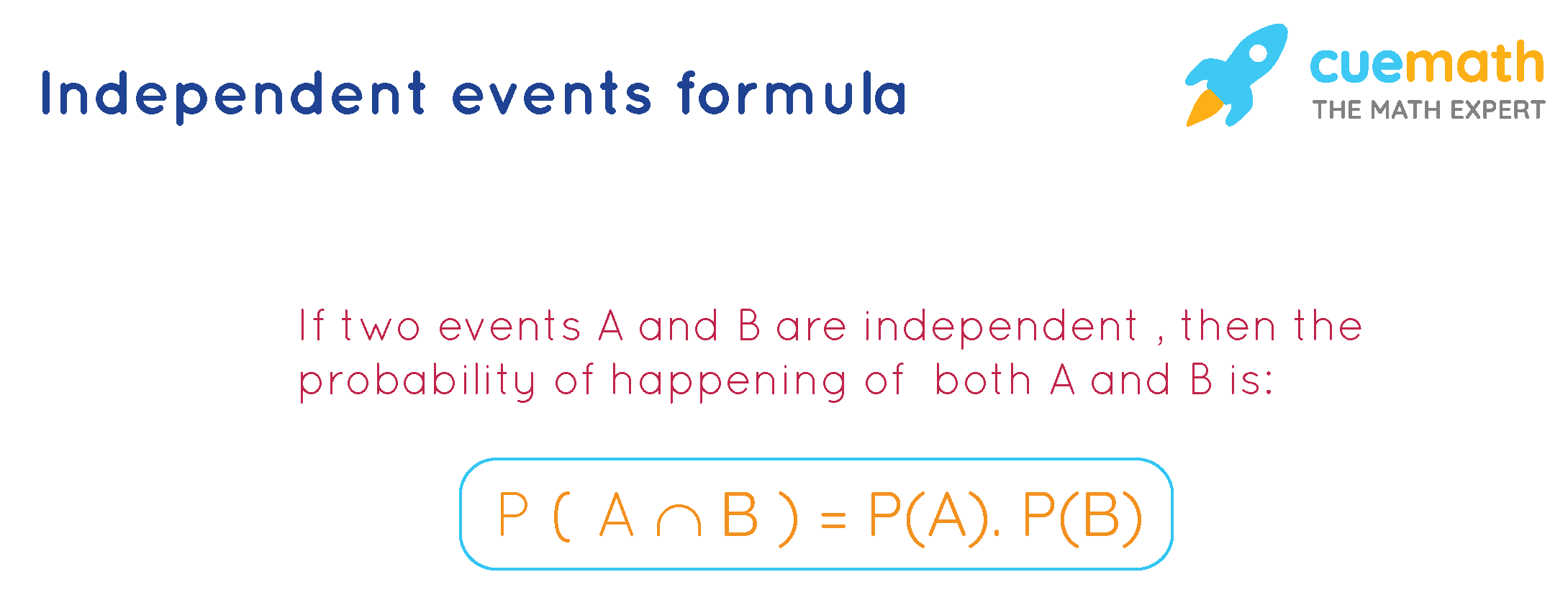# Independent Events Formula

Two events are said to be independent if the occurrence of one of the events doesn't affect the probability of the other event, and the two events are said to be dependent if they are NOT independent. Let us learn about the independent events' formula with a few solved examples in the end. Few examples, "Two cards are drawn from a pack of cards where the first card is placed back in the pack before drawing the second card" is an independent event. "Two cards are drawn from a pack of cards where the first card is NOT placed back in the pack before drawing the second card" is a dependent event. We know that P(A | B) is the probability of event A that depends upon event B.

## What Is the Independent Events Formula?

Let us consider two events A and B. In general, we know that the probability of happening of both events A and B is: $$P(A \cap B)=p(A \mid B) p(B)=P(B \mid A) P(A)$$. Now, if A and B are independent, by the definition of independent events,

• ### P(B | A) = P (B) (as B doesn't depend on A)

Thus, when the events are independent, the probability of happening of both events A and B becomes:

### $P(A \cap B)=p(A ) p(B)=P(B) P(A)$

Thus,## Solved Examples Using Independent Events Formula

### Example 1: When a die is thrown twice, find the probability of getting two 4s. Solve by using independent event formula.

Solution:

To find: Finding the probability of getting two 4s.

Let A and B be the events of getting a 4 when the die is thrown for the first and the second time respectively. Then:

P(A) = 1 / 6

P(B) = 1 / 6

We know that A and B are independent events here.

Using the formula of the independent event:

P(A ∩ B) = P(A) × P(B)

P(A ∩ B) = $$\dfrac 1 6 \cdot \dfrac 1 6 = \dfrac 1 {36}$$

Answer: The probability of getting two 4s = 1 / 36.

### Example 2: A card is chosen at random from a deck of 52 cards. It is then replaced before the second card is chosen. What is the probability of choosing a king and then a red card? Solve by using independent event formula.

Solution:

To find: The probability of choosing a king and then a red card.

Let A and B be the events of choosing a king and a red card respectively.

We know that:

P(A) = 4 / 52 = 1 / 13 (as there are 4 kings out of 52 cards)

P(B) = 26 / 52 = 1 / 2 (as there are 26 red cards out of 52 cards)

Since the first card is placed back in the deck before the second card is chosen, A and B are independent.

Using the formula of the independent event:

P(A ∩ B) = P(A) × P(B)

P(A ∩ B) = $$\dfrac 1 {13} \cdot \dfrac 1 2 = \dfrac 1 {26}$$

Answer: The probability of choosing a king and then a red card = 1 / 26.

Learn from the best math teachers and top your exams

• Live one on one classroom and doubt clearing
• Practice worksheets in and after class for conceptual clarity
• Personalized curriculum to keep up with school